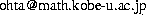$BB@ED(B $BBY9-(B $B?@8MBg3XBg3X1!M}3X8&5f2J(B $B?t3X@l96(B $B1~MQ?tM}9V:B(B $B=Z65 $B7W;;?tM}650i8&5fJ,Ln(B E-mail:Tel: 078-803-6468 $B8&5f%F!<%^(B

$BHs@~7A2D@QJ,7O!"FC$K%=%j%H%sJ}Dx<07O$N2r6u4V$NBe?t9=BN8&5f!"(B $B5Z$S$=$l$i$NJ*M}3X!&9)3X$KBP$9$k1~MQ$N8&5f(B

$B8&5f6H@S(B 1. K. Maruno and Y. Ohta, Casorati Determinant Form of Dark Soliton Solutions of the Discrete Nonlinear Schr\"odinger Equation, J. Phys. Soc. Jpn. {\bf 75}, No. 5 (2006) 054002-1--054002-10. 2. Y. Ohta, Discretization of a Sine-Gordon Type Equation, NATO Science Series II: Mathematics, Physics and Chemistry, {\bf 201}, Eds. L. D. Faddeev, P. van Moerbeke and F. Lambert, (Springer, Dordrecht, 2006) 225--230. 3. K. M. Tamizhmani, B. Grammaticos, A. Ramani, Y. Ohta and T. Tamizhmani, Similarity Reductions of Hirota Bilinear Equations and Painlev\'e Equations, NATO Science Series II: Mathematics, Physics and Chemistry, {\bf 201}, Eds. L. D. Faddeev, P. van Moerbeke and F. Lambert, (Springer, Dordrecht, 2006) 313--323. 4. A. Ramani, Y. Ohta and B. Grammaticos, Discrete Systems Related to the Sixth Painlev\'e Equation, J. Phys. A: Math. Gen. {\bf 39}, No. 39 (2006) 12167--12178. 5. C.-X. Li and Y. Ohta, Gram-type Pfaffian solution to the coupled discrete KP equation, J. Phys. A {\bf 38} No. 5 (2005) 1089--1095. 6. K. Kajiwara, T. Masuda, M. Noumi, Y. Ohta and Y. Yamada, Construction of Hypergeometric Solutions to the$q$-Painlev\'e Equations, Int. Math. Res. Not. No. 24 (2005) 1439--1463. 7. K. Kajiwara, T. Masuda, M. Noumi, Y. Ohta and Y. Yamada, Cubic Pencils and Painlev\'e Hamiltonians, Funkcial. Ekvac. {\bf 48} No. 1 (2005) 147--160. 8. M. Nishizawa, Y. Ohta and S. Tsujimoto, Some Aspects of the Toda Molecule, Glasgow Math. J. {\bf 47A} (2005) 169--176. 9. K. Kajiwara, T. Masuda, M. Noumi, Y. Ohta and Y. Yamada, Hypergeometric Solutions to the$q$-Painlev\'e Equations, Int. Math. Res. Not. No. 47 (2004) 2497--2521. 10. Y. Ohta, Special Solutions of Discrete Integrable Systems, {\it Discrete Integrable Systems}, Lecture Notes in Phys. {\bf 644} (Springer, Berlin, 2004) 57--83. 11. Y. Ohta, Discretization of Toroidal Soliton Equations, J. Nonlinear Math. Phys. {\bf 10} Suppl. 2 (2003) 143--148. 12. C. Gilson, J. Hietarinta, J. Nimmo and Y. Ohta, Sasa-Satsuma Higher-Order Nonlinear Schr\"odinger Equation and Its Bilinearization and Multisoliton Solutions, Phys. Rev. E {\bf 68} No.1 (2003) 016614. 13. K. Kajiwara, T. Masuda, M. Noumi, Y. Ohta and Y. Yamada,${}_{10}E_9$Solution to the Elliptic Painlev\'e Equation, J. Phys. A {\bf 36} No. 17 (2003) L263--L272. 14. K. Maruno, Y. Ohta and N. Joshi, Exact Localized Solutions of Quintic Discrete Nonlinear Schr\"odinger Equation, Phys. Lett. A {\bf 311} No. 2-3 (2003) 214--220. 15. B. Grammaticos, A. Ramani and Y. Ohta, A Unified Description of the Asymmetric$q$-P$_{\rm V}$and$d$-P$_{\rm IV}\$ Equations and Their Schlesinger Transformations, J. Nonlinear Math. Phys. {\bf 10}, No. 2 (2003) 215--228.

16. T. Takenawa, M. Eguchi, B. Grammaticos, Y. Ohta, A. Ramani and J. Satsuma, The Space of Initial Conditions for Linearizable Mappings, Nonlinearity {\bf 16}, No. 2 (2003) 457--477.

17. T. Masuda, Y. Ohta and K. Kajiwara, A Determinant Formula for a Class of Rational Solutions of Painlev\'e V Equation, Nagoya Math. J. {\bf 168} (2002) 1--25.

18. Y. Ohta, A. Ramani and B. Grammaticos, Elliptic Discrete Painlev\'e Equations, J. Phys. A {\bf 35}, No. 45 (2002) L653--L659.

19. K. Kimura, H. Yahagi, R. Hirota, A. Ramani, B. Grammaticos and Y. Ohta, A New Class of Integrable Discrete Systems, J. Phys. A {\bf 35}, No. 43 (2002) 9205--9212.

20. A. Ramani, A. S. Carstea, B. Grammaticos and Y. Ohta, On the Autonomous Limit of Discrete Painlev\'e Equations, Phys. A {\bf 305}, No. 3-4 (2002) 437--444.